## Example Questions

← Previous 1 3 4 5 6 7 8 9

### Example Question #1 : How To Find An Angle In A Right Triangle

The measure of 3 angles in a triangle are in a 1:2:3 ratio. What is the measure of the middle angle?

30
90
60
45
Explanation:

The angles in a triangle sum to 180 degrees. This makes the middle angle 60 degrees.

### Example Question #1 : How To Find An Angle In A Right Triangle

A 17 ft ladder is propped against a 15 ft wall. What is the degree measurement between the ladder and the ground?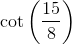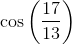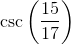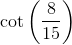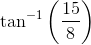Explanation:

Since all the answer choices are in trigonometric form, we know we must not necessarily solve for the exact value (although we can do that and calculate each choice to see if it matches). The first step is to determine the length of the ground between the bottom of the ladder and the wall via the Pythagorean Theorem: "x+ 152 = 172"; x = 8. Using trigonometric definitions, we know that "opposite/adjecent = tan(theta)"; since we have both values of the sides (opp = 15 and adj = 8), we can plug into the tangential form tan(theta) = 15/8. However, since we are solving for theta, we must take the inverse tangent of the left side, "tan-1". Thus, our final answer is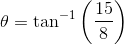### Example Question #1 : Triangles

What is the sine of the angle between the base and the hypotenuse of a right triangle with a base of 4 and a height of 3?Explanation:

By rule, this is a 3-4-5 right triangle. Sine = (the opposite leg)/(the hypotenuse). This gives us 3/5.

### Example Question #1 : Triangles

In a right triangle ABC, the measure of angle C is greater than 60 degrees. Which of the following statements could describe the measures of angles A and B?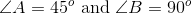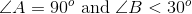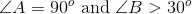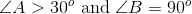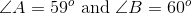Explanation:

Given that it is a right triangle, either angle A or B has to be 90 degrees. The other angle then must be less than 30 degrees, given that C is greater than 60 because there are 180 degrees in a triangle.

Example:

If angle C is 61 degrees and angle A is 90 degrees, then angle B must be 29 degrees in order for the angle measures to sum to 180 degrees.

### Example Question #5 : Right Triangles

Right triangle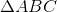has angles with a ratio of with a ratio of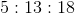. What is the smallest angle in the triangle?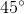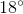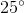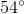Explanation:

Solving this problem quickly requires that we recognize how to break apart our ratio.

The Triangle Angle Sum Theorem states that the sum of all interior angles in a triangle is. Additionally, the Right Triangle Acute Angle Theorem states that the two non-right angles in a right triangle are acute; that is to say, the right angle is always the largest angle in a right triangle.

Since this is true, we can assume thatis represented by the largest number in the ratio of angles. Now consider that the other two angles must also sum to. We know therefore that the sum of their ratios must be divisible byas well.

Thus,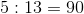.

To find the value of one angle of the ratio, simply assign fractional value to the sum of the ratios and multiply by.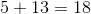, so: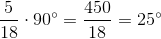Thus, the shortest angle (the one represented byin our ratio of angles) is.

### Example Question #1 : Triangles

Right trianglehas an acute angle measuring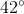. What is the measure of the other acute angle?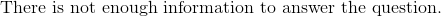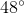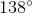Explanation:

The Triangle Angle Sum Theorem states that the sum of all interior angles in a triangle must be. We know that a right triangle has one angle equal to, and we are told one of the acute angles is.

The rest is simple subtraction: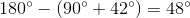Thus, our missing angle is.

### Example Question #1 : Triangles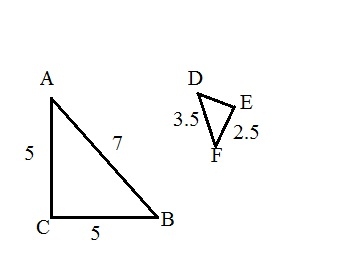is similar to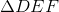. What is the measurement of segment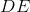?Explanation:

The word similar means, comparable in measurement, but not equal. The best sides way to compare these two triangles is by looking at the diagonal side of the triangle since it cannot be mistaken for any of the other sides of either triangle. If the larger triangle has a measurement of 7 and the smaller triangle has a measurement of 3.5 for their diagonal sides, then that means the ratio of the larger triangle to the smaller one is.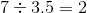This means that segment EF must be similar to segment AC (look at the orientation). So, since segment DE is similar to segment CB, divide 5 by two to get your answer.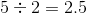### Example Question #61 : Geometry

There are two similar right triangles. One has side lengths of 6, 8, and 10. The other has side lengths of 24, and 18. What is the length of the missing side?Explanation:

1. Since the two triangles are similar, find the ratio of the two triangles to each other:

In this case, both triangles are multiples of a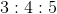special right triangle.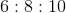and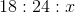The ratio of the triangles is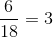2. Use the ratio you found to solve for, or the length of the missing side: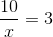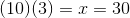### Example Question #9 : Right Triangles

There are two right triangles, Triangle A and Triangle B. Triangle A has sides with lengths of 3, 4, and 5 and Triangle B has only two given side lengths: 3 and 4. The length of the hypotenuse is not given. Are these two triangles congruent?

Yes, because the missing hypotenuse length is 4.

No, because you do not know the angle measures.

Yes, because the missing hypotenuse length is 5.

It is impossible to say solely based on the provided information.

Yes, because the missing hypotenuse length is 5.

Explanation:

1. Find the length of the missing hypotenuse:

You can do this one of two ways:

1) Using the special 3-4-5 right triangle, you can infer that the missing hypotenuse is 5.

2) By using the Pythagorean Theorem, you can solve for the length of the hypotenuse:In this case: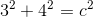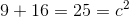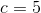2. Using the meaning of congruent (the exact same three angles and sides), determine if these two triangles fit this meaning:

They do, because both are 3-4-5 right triangles, and thus must have corresponding and equal angles due to trigonometric properties.

### Example Question #5 : How To Find The Area Of A Right Triangle

The ratio for the side lengths of a right triangle is 3:4:5. If the perimeter is 48, what is the area of the triangle?

50

240

108

48

96

96

Explanation:

We can model the side lengths of the triangle as 3x, 4x, and 5x. We know that perimeter is 3x+4x+5x=48, which implies that x=4. This tells us that the legs of the right triangle are 3x=12 and 4x=16, therefore the area is A=1/2 bh=(1/2)(12)(16)=96.

← Previous 1 3 4 5 6 7 8 9

### All ACT Math Resources